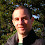# logji

noodling towards a functional brain

## Thursday, July 29, 2010

### A monad for failure-tolerant computations.

I was working on a problem today where some of the computations could fail, but degrade gracefully while providing information about how exactly they failed so that clients could choose whether or not to use the result. This is what I came up with:
```/**
* A monad used to entrain computations on a value that might fail.
* This is distinct from Either in that it allows computations to continue
* in the presence of errors, with the possibility of a degraded result.
* The user of the result can then decide its suitability for use based upon
* any errors that might be returned. Errors must form a semigroup.
*/
sealed trait Attempt[E, +A] {
def value: A
def map[B](f: A => B): Attempt[E, B]
def flatMap[B](f: A => Attempt[E, B]): Attempt[E, B]

def error: Option[E]
def either: Either[E, A]
}

case class Success[E, +A](value: A) extends Attempt[E, A] {
def map[B](f: A => B): Attempt[E, B] = Success(f(value))
def flatMap[B](f: A => Attempt[E, B]): Attempt[E, B] = f(value)
def error = None
def either = Right(value)
}

case class Failure[E, +A](err: E, value: A)(implicit append: (E, E) => E) extends Attempt[E, A] {
def map[B](f: A => B): Attempt[E, B] = Failure(err, f(value))
def flatMap[B](f: A => Attempt[E, B]): Attempt[E, B] = f(value) match {
case Failure(e, b) => Failure(append(e, err), b)
case Success(b)   => Failure(err, b)
}

def error = Some(err)
def either = Left(err)
}
```
Pretty trivial, but maybe occasionally useful. Here's how it looks in my code:
```  def mergeComponents(f: String => JSONReport): Attempt[List[String], JSONReport] = {
components.map(f).map(_.mergeComponents(f)).foldLeft[Attempt[List[String], JSONReport]](Success(this)) {
(result, comp) => for {
cur <- result
jr  <- comp
properties     <- mergeProperties(cur, jr)
queries        <- mergeQueries(cur, jr)
dataAliases    <- mergeAliases(cur, jr, queries.values)
dataTransforms <- mergeTransforms(cur, jr, dataAliases.values)
dataMailers    <- mergeMailers(cur, jr, dataTransforms.values)
} yield {
JSONReport(
cur.reportId, cur.active, cur.version, properties,
queries, dataAliases, dataTransforms, dataMailers,
cur.dataRange, Nil
)
}
}
```

Here each of the various mergeX methods return an Attempt[List[String], X] where X is something needed to build the ultimate report. Here I'm just aggregating lists of strings describing the errors, but of course any type for which (E, E) => E is defined would work.

Attempt. For all those times where you want a monad that says, "Hey, I maybe couldn't get exactly what you asked for. Maybe it's little broken, maybe it won't work right, but this the best I could do. You decide."

EDIT:

After a bit of thinking, I realized that this monad is really more general than being simply related to success or failure - it simply models a function that may or may not produce some additional metadata about its result. Then a lightbulb went off, and quick google search confirmed... yup, I just reinvented the writer monad. It's not *exactly* like Writer, because it just requires a semigroup for E instead of a monoid, and the presence of a "log" is optional, so maybe it's better suited than Writer for a few instances.

The one really nice thing about rediscovering well-known concepts is that doing the derivation for yourself, in the context of some real problem, gives you a much more complete understanding of where the thing you reintevented is applicable!

Inspired by michid's example in the comments below, here's a simpler example that demonstrates some utility his doesn't quite capture.

```implicit val append = (l1: List[String], l2: List[String]) => l1 ::: l2

def succ(s: String) = Success[List[String], String]("result: success")
val fail: String => Attempt[List[String], String] = {
var count = 0
(s: String) => {
count += 1
Failure(List("failure " + count), s + " then failure")
}
}

val r = for {
x <- succ("here we go!")
y <- fail(x)
z <- fail(y)
} yield z

println(r)
```
This results in:
```Failure(List(failure 2, failure 1),result: success then failure then failure)
```

1.Hey Kris, scalaz's validations may be what you're looking for here:

http://github.com/scalaz/scalaz/blob/master/core/src/main/scala/scalaz/Validation.scala

2.Hi, Tom. Validations isn't quite the same thing; it's rather more similar to Either in that in the event of a failure, it doesn't retain both the error and a degraded result value.

3.Hey Kris,
Looks interesting! Could you please provide a example for dummies?
Thanks,
Heiko

4.Nice! Here a simpler example which I've come up with:

val s: Attempt[List[String], String] = Success("result: success")
val f1: Attempt[List[String], String] = Failure("fail 1"::Nil, "result: failure 1")
val f2: Attempt[List[String], String] = Failure("fail 2"::Nil, "result: failure 2")

val r =
for {
x <- s
y <- f1
z <- f2
}
yield (x, y, z)

println(r)

This prints:

Failure(List(fail 2, fail 1),(result: success,result: failure 1,result: failure 2))

5.Anonymous9:31 AM

Yeah, it's Writer specialized to the monoid Option[S] where S is a semigroup. But you could simplify your definition to allow any monoid, thus becoming exactly the writer monad.

case class Attempt[E:Monoid, +A](err: E, val: A)